# const - JS | lectureHow JavaScript Works Behind the Scenes

The this Keyword in Practice

Let's now see the rules of how the this keyword is defined in action.

Let's start by looking at the this keywords outside of any function, i.e., the global scope.

script.js
``console.log(this)``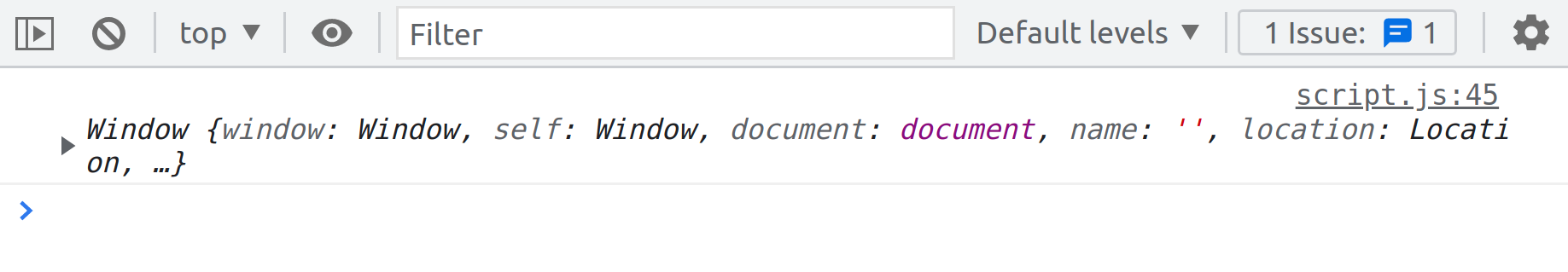We see that the this keyword in the global scope is simply the `window` object in our browser console.

Now, let's do the same, but inside of just a regular function call.

script.js
``````const calcAge = function (birthYear) {
console.log(2025 - birthYear);
console.log(this);};

calcAge(1996);``````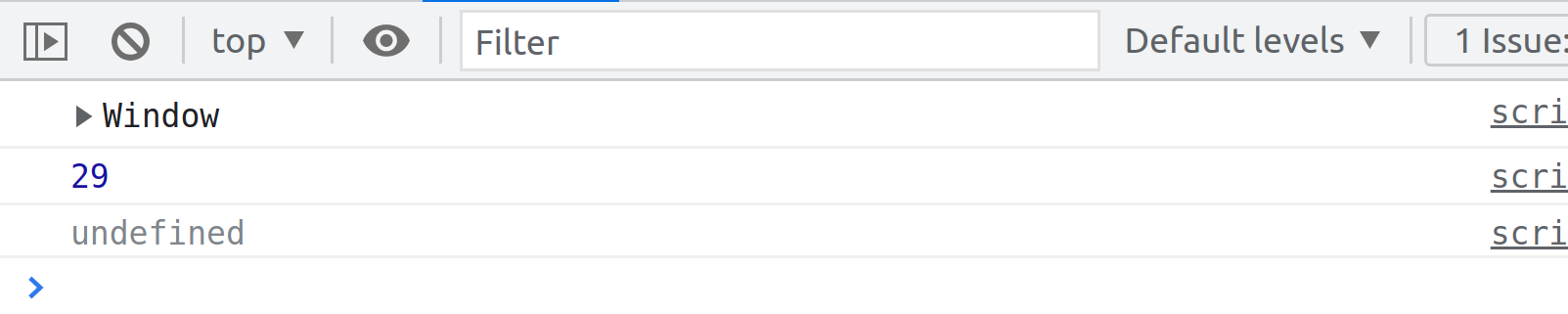From the result, we can see that the this keyword is `undefined`. That's because we are in strict mode. Remember that in sloppy mode, it would be the global `window` object.

Next, let's see what happens if our calcAge function is an arrow function.

script.js
``````const calcAgeArr = (birthYear) => {
console.log(2025 - birthYear);
console.log(this);};

calcAgeArr(1996);``````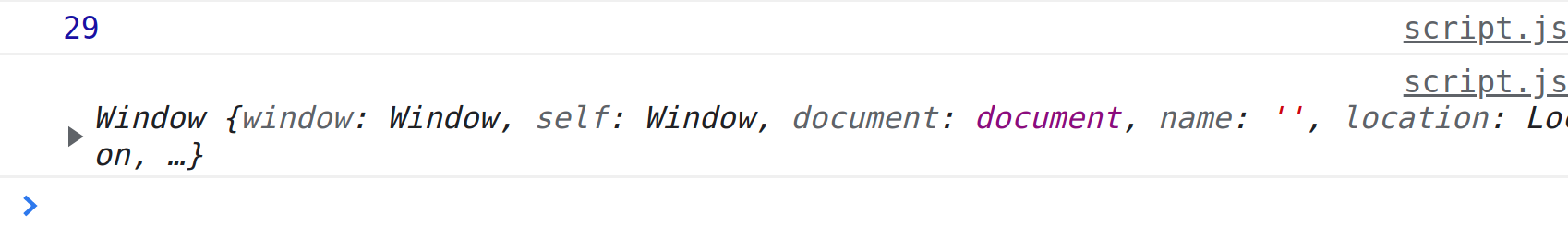We can see from the result that the value of the this keyword in an arrow function is the `window` object. It might be a bit weird, and you may ask yourself, why is the this keyword `undefined` in the `calcAge` function but `window` in the `calcAgeArr` function? It is because the arrow function does not get its own this keyword. Instead, the arrow function simply uses the lexical this keyword, which means that it uses the this keyword of its parent function or its parent's scope.

In this case, the lexical this keyword or the this keyword in the parent's scope is `window` because `window` is the this keyword in the global scope. That's why we started this lecture by logging the value of this.

Next up, let's try to use the this keyword inside a method.

script.js
``````const john = {
year: 1991,
calcAge: function () {
console.log(this)};

john.calcAge();``````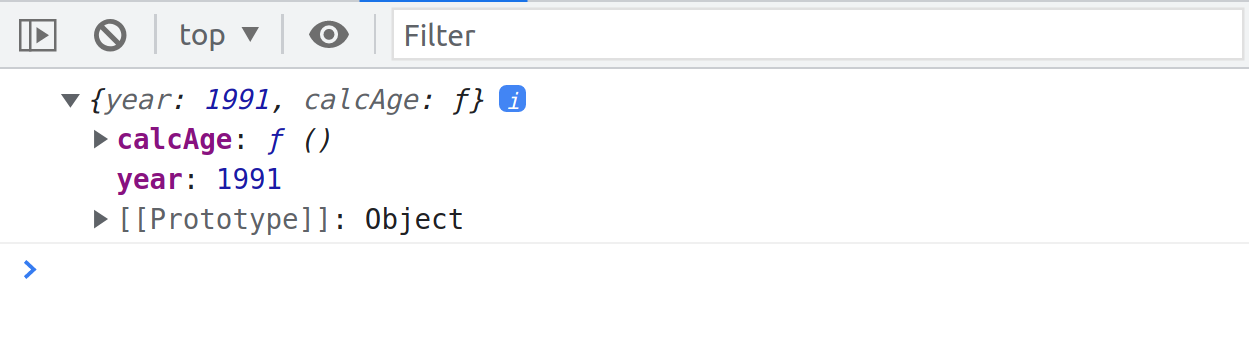From our console, we can see that the value of the this keyword in the `calcAge` method is the `john` object. That's exactly the rule that I told you in the previous lecture. When we have a method call, the this keyword inside the method will be the object calling the method.

In this case, that's the `john` object. So when we do `john.calcAge()`, `john` is basically the owner of the method. That's another way of saying it and, therefore, inside of `calcAge`, this is `john`.

We can then use that to our advantage to calculate the age based on the data that is already in the `john` object.

script.js
``````const john = {
year: 1991,
calcAge: function () {
console.log(this)
console.log(2025 - this.year);};

john.calcAge();``````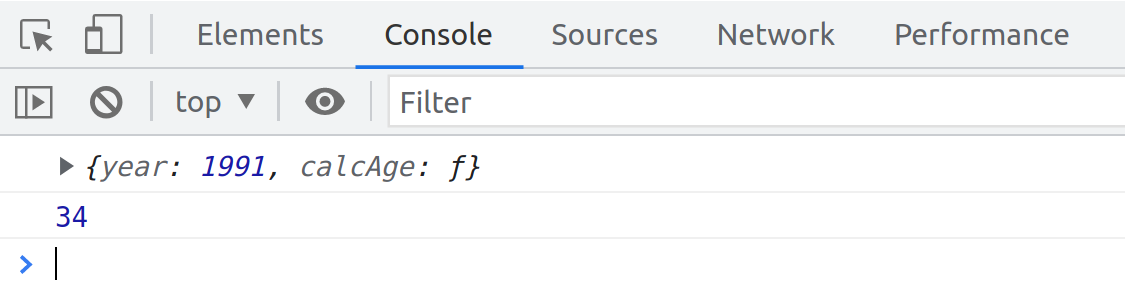That's one of the big use cases for the this keyword because we don't have to pass in data in our `calcAge` function anymore. That is, instead of doing something like this:

script.js
``````// JS Code

john.calcAge(1991);``````

We can simply use the birth year already in the object itself. And so we can use the this keyword to reference that object.

Now let's move on just to one final example in this lecture. I keep saying that the this keyword will point to the object calling the method, right? And that means that the this keyword will not simply point at the object in which we wrote the method.

In the above example, we wrote the `calcAge` method inside of the `john` object, and so we might think that it is the reason why the this keyword points to `john`, but that is not true.

Let me know, show you why that is. Let's create a new object called `mary`.

script.js
``````const mary = {
year: 1998,
};``````

Next, remember that a function is just a value. Therefore we can do this.

script.js
``````const mary = {
year: 1998,
};

// It prevents duplicating methods
mary.calcAge = john.calcAge;``````

We are simply copying the `calcAge` method from `john` to `mary`. If we look at `mary` in the console, we see that the `calcAge` function is also present. And of course it's still also in `john`, but now we copied it from one object to the other, and this is called method borrowing.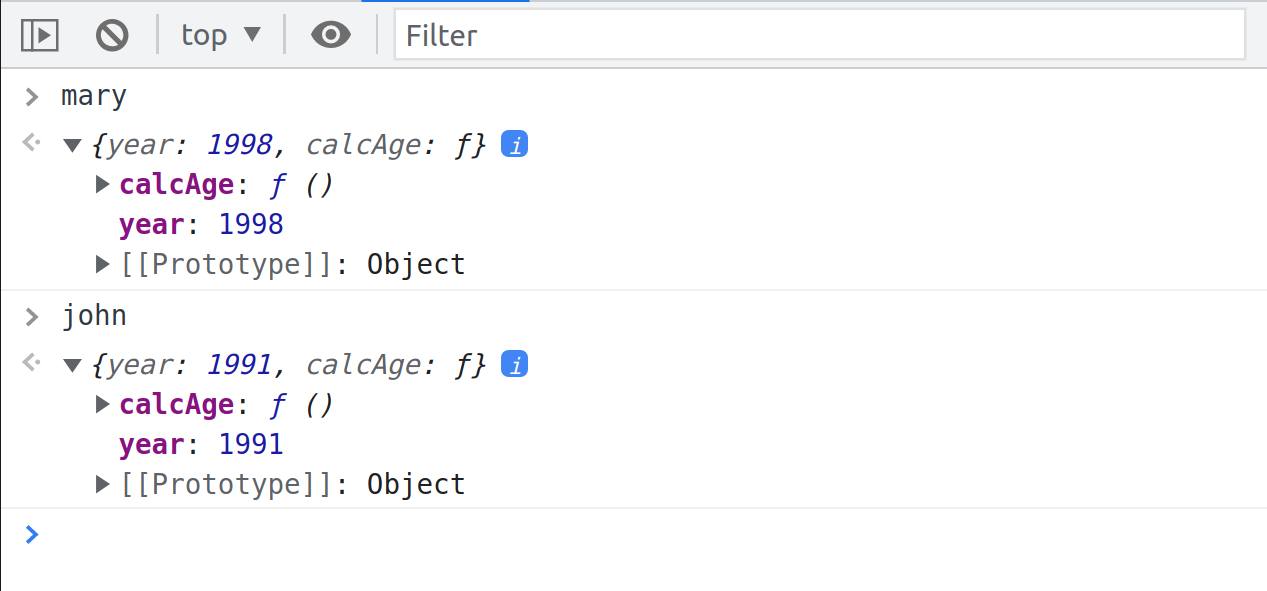Let's now call the method on `mary`

script.js
``mary.calcAge();``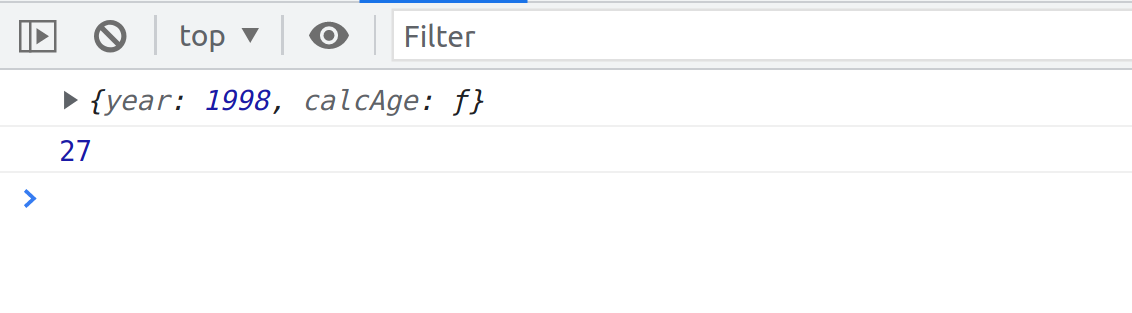From the console, we can see that the age of mary gave us the correct result of `27`, which is exactly 2025 minus 1998. This means that when doing `mary.calcAge()`, the this keyword does now actually point to `mary`. We can also see that as we log the this keyword in the console, we see that it's actually the `mary` object because of the birth year which is `1998`.

This proves exactly what I was telling you before: the this keyword always points to the object calling the method.

Even though the method is written inside the `john` object, the this keyword will still point to `mary` if it is `mary` calling the method. That's really important to understand, and it's the reason why I said in the last lecture that the this keyword is really dynamic. It's not static, and it depends on how the function is called.

We can take this even further by basically completely taking the function out of the `john` object.

Let's create a simple variable called `func` for function and assign it the value of `john.calcAge`.

script.js
``````/**
* We are just copying the function calcAge
* into the variable func. We are NOT calling it.
*/
const func = john.calcAge``````

Now the question is, what implications will this have for the "this" keyword? Let's try to call the `func` function, which is the code of `john.calcAge`.

script.js
``````const func = john.calcAge

func()``````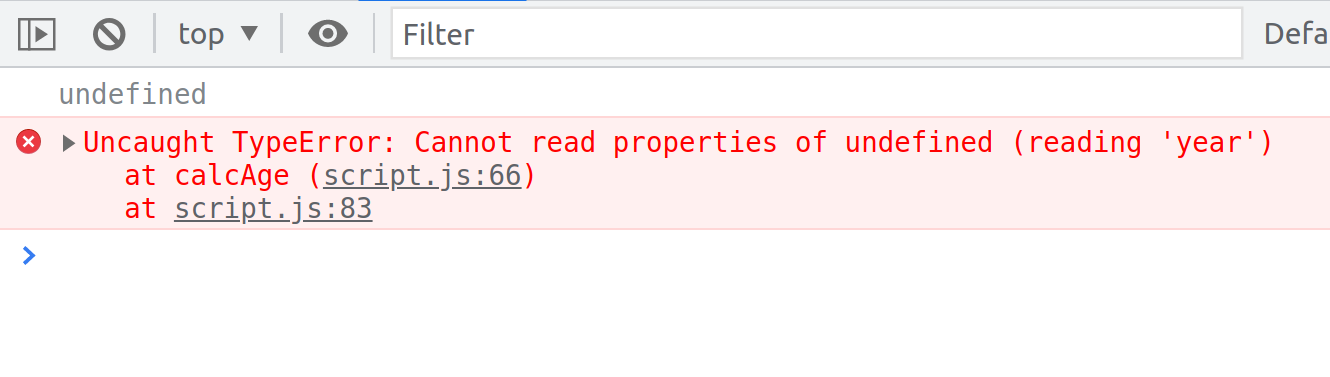We can see from the result that the this keyword is `undefined` and we are also getting an error saying that it cannot read `year` of `undefined`. That error is coming from here:

script.js
``console.log(2025 - this.year);``

Because the this keyword is now `undefined`, and so `undefined.year` does, of course, not exist. This happens because the `func` function (`func()`) is now just a regular function call. It is not attached to any object. There is no owner of the `func` function, and therefore it is just a regular function call just like when we had this:

script.js
``calcAge(1996);``

And so therefore, the this keyword is then also `undefined`.

Again, that's a subtle difference in our code, but it makes all the difference in the result that we then get. And for sure, the this keyword is a subject that confuses many beginners in JavaScript, but hopefully, this lecture and the previous one made it a little bit more clear.

We didn't talk about the event handler functions here that I also showed you in the last lecture. I'm leaving that for the advanced DOM section where we will come back to this, but for now, in the next lecture, we need to take a closer look at regular functions versus arrow functions.